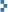Code snippet: all paths between two nodes in a graph

January 13th, 2013

An adapted version (of code found at Python patterns) for finding all the paths between two given nodes in a NetworkX graph, with the additional limit of length k (where k is the maximum number of edges in the path).

```def find_all_paths_lim(graph, start, end, k, path=[]):
path = path + [start]
if start == end:
return [path]
if not start in graph:
return []
paths = []
for node in graph[start]:
if node not in path:
if len(path) < k+1:
newpaths = find_all_paths_lim(graph, node, end, k, path)
for newpath in newpaths:
paths.append(newpath)
return paths```

So, for example:

```import networkx as nx
G=nx.Graph()
`find_all_paths_lim(G,"beans","black pudding",3)`

gives you

`[['beans', 'egg', 'spam', 'black pudding']]`

and

`find_all_paths_lim(G,"beans","black pudding",4)`
gives you
`[['beans', 'egg', 'spam', 'white pudding', 'black pudding'],`
`['beans', 'egg', 'spam', 'black pudding']]`

Note: loops are omitted and works on MultiDiGraphs (i.e. directed and multi-labeled NetworkX graphs).